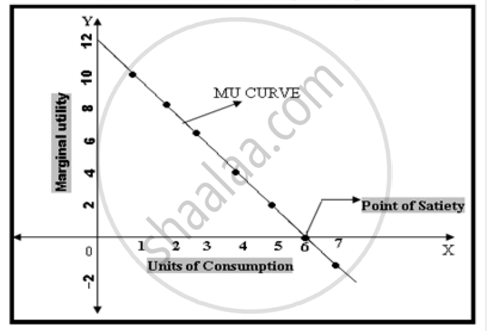# Explain the Law of Diminishing Marginal Utility. - Economics

Explain the law of diminishing marginal utility.

#### Solution

Statement of the Law:-

The law of diminishing Marginal utility states that (other thing being equal) s the number of units consumed of commodity increases, the marginal utility of that commodity diminishes. In other words, as the consumption of good increases, the marginal utility derived from successive units of a given commodities goes on diminishing.

Definition:-

In he words of Marshal the Law states that, “The additional benefit which a person derives from a given increase of his stock of a thing, diminishes with every increase in the stock that he already has”

The law of diminishing Marginal Utility can be explained with the help of a schedule and a diagram.

 Units of Consumption Total Utility (T.U.) Marginal Utility () 1 10 10 2 18 8 3 24 6 4 28 4 5 30 2 6 30 0 7 28 -2

The schedule shows that with every increase in the units of Consumption, the total utility is increasing. It reaches Maximum with the 5th & 6th unit and remains the same, but with 7th unit the total utility decreases from 30-28.

The Marginal Utility can be derived from total utility. It is observed the Marginal Utility is falling continuously. It reaches zero and then become negative.

The Marginal Utility is Zero when total utility is Maximum and Marginal utility is negative when total utility is falling.In the above diagram

X- Axis represents units of consumption and Y-Axis represents Marginal Utility. Various points from the table are plotted on graph. Join those points we can get a curve known as Marginal utility curve. The curve slopes down wards from left to right. It touches X- axis and becomes negative. It is observed from the diagram that at 6th unit of consumption the marginal utility becomes zero, when the total utility becomes maximum. It is the point of Satiety i.e. the want is completely satisfied and its intensity is nil. With the 7th unit of consumption the total utility started falling and the Marginal Utility becomes negative.

Concept: Diminishing Marginal Utility
Is there an error in this question or solution?
2014-2015 (March)

Share